# CLASS 9 SCIENCE NCERT SOLUTION FOR CHAPTER – 3 Atoms and Molecules

## Atoms and Molecules

INTEXT Questions

Question 1.
In a reaction, 5.3 g of sodium carbonate reacted with 6 g of ethanoic acid. The products were 2.2 g of carbon dioxide, 0.9 g of water and 8.2 g of sodium ethanoate. Show that these observations are in agreement with the law of conservation of mass.

Sodium carbonate + ethanoic acid → sodium ethanoate + carbon dioxide + water

Solution:
Total mass of reactants = mass of sodium carbonate + mass of ethanoic acid
= 5 – 3 g + 6 g = 11.3 g
Total mass of products = mass of sodium ethanoate + mass of carbon dioxide + mass of water
= 8.2 g + 2.2 g + 0.9 g = 11.3 g
Thus, the mass of reactants is equal to the mass of products, therefore the observations are in agreement with the law of conservation of mass.

Question 2.
Hydrogen and oxygen combine in the ratio of 1:8 by mass to form water. What mass of oxygen gas would be required to react completely with 3 g of hydrogen gas?

Solution:
∵ 1 g of hydrogen reacts with = 8 g of oxygen
∴ 3 g of hydrogen reacts with = 8 × 3 = 24 g of oxygen
Thus, 24 g of oxygen gas would be required to react completely with 3 g of hydrogen gas.

Question 3.
Which postulate of Dalton’s atomic theory is the result of the law of conservation of mass?

Solution:
The postulate that “atoms can neither be created nor destroyed in a chemical reaction” is the result of the law of conservation of mass.

Question 4.
Which postulate of Dalton’s atomic theory can explain the law of definite proportions?

Solution:
The postulate that “A chemical compound always consists of the same elements combined together in the same proportion by mass” is the law of definite proportions.

Question 5.
Define the atomic mass unit.

Solution:
Atomic mass unit is defined as the mass unit equal to exactly one-twelfth (1/12th) of the mass of one atom of carbon-12. It is denoted by u (unified mass).
i.e. 1 u = 1.66 × 10-24 g

Question 6.
Why is it not possible to see an atom with naked eyes?

Solution:
It is not possible to see an atom with naked eye because of its extremely small size (atomic radius is of the order of 10-10 m).

Question 7.
Write down the formula of

1. sodium oxide’
2. aluminium chloride
3. sodium sulphide
4. magnesium hydroxide.

Solution:

Sodium oxideAluminium chlorideSodium sulphide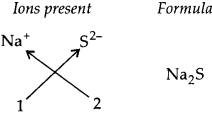Magnesium hydroxideQuestion 8.
Write down the names of compounds represented by the following formulae:

1. AI2(S04)3
2. CaCI2
3. K2S04
4. KNO3
5. CaCO3

Solution:

(i) Aluminium sulphate
(ii) Calcium chloride
(iii) Potassium sulphate
(iv) Potassium nitrate
(v) Calcium carbonate

Question 9.
What is meant by the term chemical formulae?

Solution:
The chemical formula of a compound is a symbolic representation of its composition. e.g., formula of calcium oxide.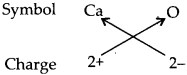Thus, chemical formula of calcium oxide is CaO.

Question 10.
How many atoms are present in a

(i) H2S molecule and
(ii)${ PO }_{ 4 }^{ 3- }$ ion?

Solution:
(i) 3 atoms because H2S molecule has two atoms of hydrogen and one atom of sulphur.
(ii) 5 atoms because${ PO }_{ 4 }^{ 3- }$ ion has one atom of phosphorus and four atoms of oxygen.

Question 11.
Calculate the molecular masses of H2, O2 Cl2, CO2, CH4 C2H6, C2H4 NH3, CH3OH.

Solution: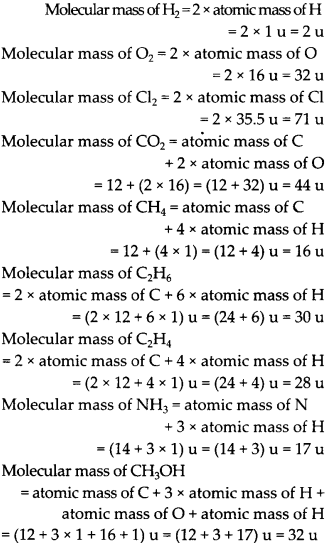Question 12.
Calculate the formula unit masses of ZnO, Na2O, K2C03. Given atomic masses of Zn = 65 u, Na = 23 u, K = 39 u,C = 12 u and,0= 16 u.

Solution:
Formula unit mass of ZnO = atomic mass of Zn + atomic mass of OQuestion 13.
If one mole of carbon atoms weighs 12 gram, what is the mass (in gram) of 1 atom of carbon?

Solution: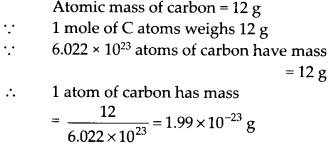Question 14.
Which has more number of atoms, 100 grams of sodium or 100 grams of iron (given atomic mass of Na = 23 u, Fe = 56 u)?

Solution:

### NCERT Exercises

Question 1.
A 0.24 g sample of compound of oxygen and boron was found by analysis to contain 0.096 g of boron and 0.144 g of oxygen. Calculate the percentage composition of the compound by weight.

Solution:
We know that, % of any element in aQuestion 2.
When 3.0 g of carbon is burnt in 8.00 g oxygen, 11.0 g of carbon dioxide is produced. What mass of carbon dioxide will be formed when 3.0 g of carbon is burnt in 50.00 g of oxygen? Which law of chemical combination will govern your answer?

Solution:

Total mass of reactants = total mass of products Hence, the law of conservation of mass is proved.
Further, it also shows carbon dioxide contains carbon and oxygen in a fixed ratio by mass, which is 3 : 8. Thus, it also proves the law of constant proportions. 3 g of carbon must also combine with 8 g of oxygen only. This means that (50 – 8) = 42 g of oxygen will remain un reacted.

Question 3.
What are polyatomic ions? Give examples.

Solution:
A polyatomic ion is a group of atoms carrying positive or negative charge.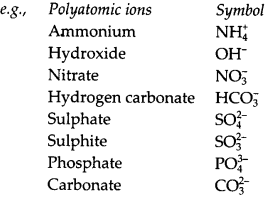Question 4.
Write the chemical formulae of the following:

(a) Magnesium chloride
(b) Calcium oxide
(c) Copper nitrate
(d) Aluminium chloride
(e) Calcium carbonate

Solution:
(a) Magnesium chloride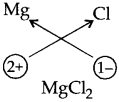(b) Calcium oxide(c) Copper nitrate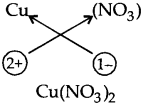(d) Aluminium chloride(e) Calcium carbonate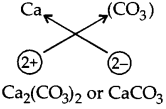Question 5.
Give the names of the elements present in the following compounds:

(a) Quicklime
(b) Hydrogen bromide
(c) Baking powder
(d) Potassium sulphate

Solution:
(a) Quick lime is CaO. Elements present are calcium and oxygen.
(b) Hydrogen bromide is HBr. Elements present are hydrogen and bromine.
(c) Baking powder is NaHC03. Elements present are sodium, hydrogen, carbon and oxygen.
(d) Potassium sulphate is K2S04. Elements present are potassium, sulphur and oxygen.

Question 6.
Calculate the molar mass of the following substances:

(a) Ethyne, C2H2
(b) Sulphur molecule, S8
(c) Phosphorus molecule, P4 (atomic mass of phosphorus = 31)
(d) Hydrochloric acid, HCI
(e) Nitric acid, HN03

Solution:

(a) Molar mass of C2H2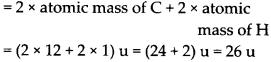(b) Molar mass of S = 8 × atomic mass of s = (8 × 32)u = 256 uQuestion 7.
What is the mass of

(a) 1 mole of nitrogen atoms?
(b) 4 moles of aluminium atoms (atomic mass of aluminium = 27)?
(c) 10 moles of sodium sulphite (Na2S03)?

Solution:Question 8.
Convert into mole.

(a) 12 g of oxygen gas
(b) 20 g of water
(c) 22 g of carbon dioxide

Solution:Question 9.
What is the mass of

(a) 0.2 mole of oxygen atoms?
(b) 0.5 mole of water molecules?

Solution:
(a) Mass of 1 mole of oxygen atom = 16 u Mass of 0.2 mole of oxygen atoms = (16 × 0.2) u = 3.2 u

(b) Mass of 1 mole of H20 molecule =Question 10.
Calculate the number of molecules of sulphur (S8) present in 16 g of solid sulphur?

Solution:
Mass of 1 mole of S8 = 8 × 32 = 256

Question 11.
Calculate the number of aluminium ions present in 0.051 g of aluminium oxide. (Hint: The mass of an ion is the same as that of an atom of the same element. Atomic mass of Al = 27 u)

Solution:
Molar mass of Al2O3 = 2 × 27 + 3 × 16#### CLASS 9 SCIENCE NCERT SOLUTION FOR CHAPTER – 4 Structure Of The Atom

Lorem ipsum dolor sit amet, consectetur adipiscing elit. Phasellus cursus rutrum est nec suscipit. Ut et ultrices nisi. Vivamus id nisl ligula. Nulla sed iaculis ipsum.

Company Name